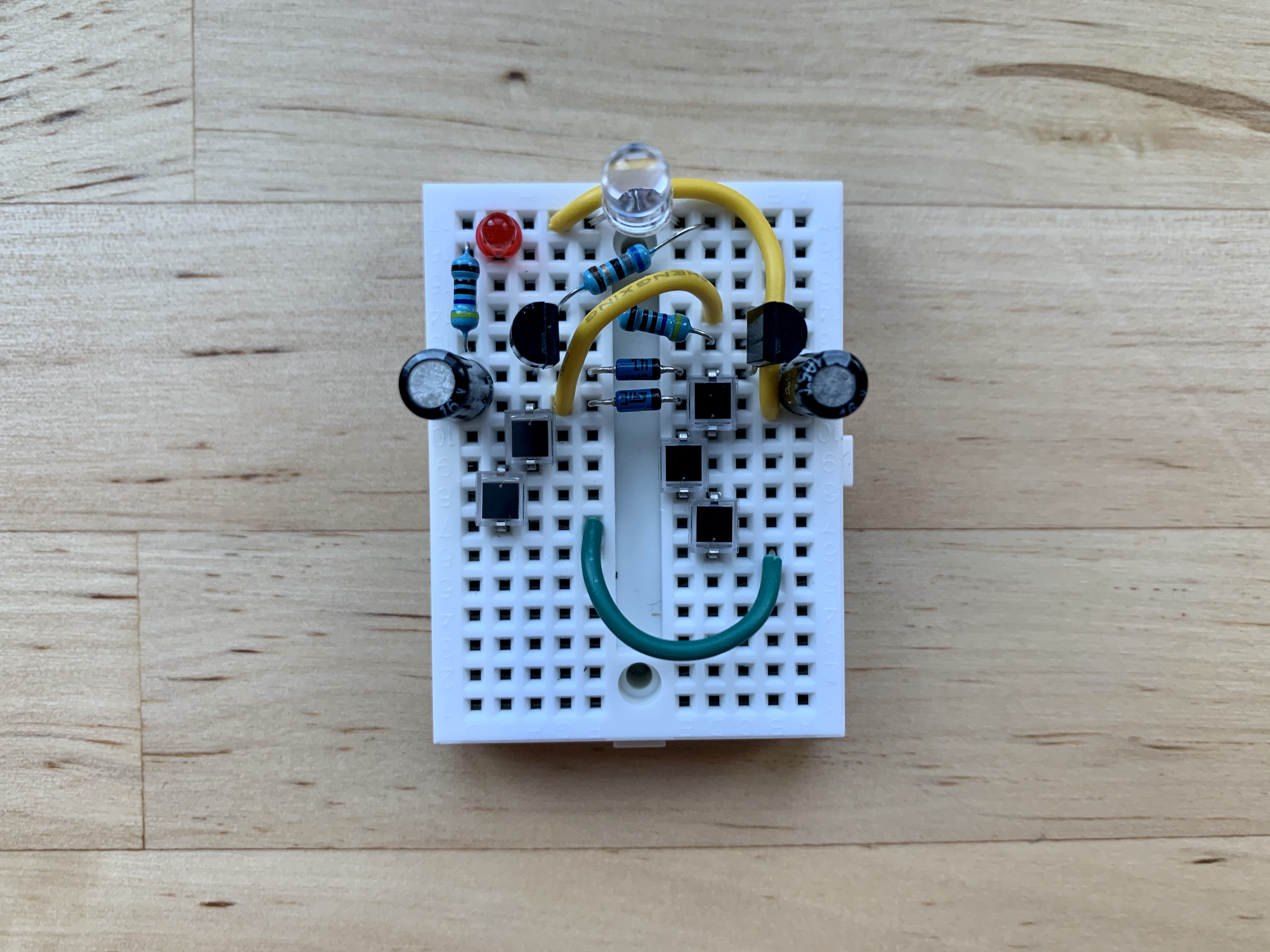# Julian Straub

## creations and thoughts

Inspired by the desire for a minimal light harvester that relies on a very small and even form-able solar cell, I recently had the idea of trying to use photo-diodes for light harvesting instead of “big” solar cells. It turns out that others have thought of this as well.To test it out, I went with the charge-pump light harvester design and adapted it to the lowest input and trigger voltage to drive a ultra-bright red LED:

• $$R_0=4.7k\Omega, R_1=4.7k\Omega, R_2=68\Omega, C_0=C_1=100\mu F$$ .
• $$D_0=D_1=$$ Schottky diode.
• $$T_0=$$ 2N3904 and $$T_1=$$ 2N3906.
• $$D_2=$$ low power red LED
• $$D_3=$$ ultra-bright red LED.
• a series of five BPW34 PIN photodiodes for roughly 2.5V.

The harvester blinks nicely at a surprisingly good frequency (relative to the more powerful solar-cell-based versions) considering the BPW34 rating of only $$50\mu A$$ photo-current.